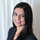Your Guide to Multivariate Linear Regressions in R

Once you’ve gotten familiar with bivariate linear regressions (one explanatory variable, and one response variable), you may want to delve into seeing how multiple explanatory variables contribute to your response. Not sure how to get started? Well you've come to the right place :) NOTE: If you are new to regressions, we highly recommend starting off with the bivariate linear regression post linked earlier for background!

The equation for a multivariate linear regression looks like this: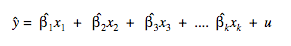y-hat is the predicted y value, each explanatory variable corresponds to one of the x's, and beta is the amount of change in y with a unit change in x, holding all other x's constant. Note, beta is also commonly written as m, and thought of as the slope of the line in a bivariate regression.

Part 1: Set Up

To begin, we will import the following libraries, which will comes useful in our multiple regression.

```library(dplyr)
library(lmtest)
library(car)
library(sandwich)```

Now normally you would be using your SQL output, but for the sake of this post we will be using the built in mtcars library and assign that to our dataframe df.

`df <- mtcars`

First, we need to explore our variables to ensure they’re suitable for the regression. Ideally, your variables should be close to normally distributed. The larger your sample size, the more “ok” it is for your sample to deviate from normality. Intuitively though, you want your variables to cover the spectrum from low to high values. In other words, you don’t want a bunch of values clustered around one extreme, and only a sparse number on the other extreme. Note, if you have any indicator variables (ex: a 0 or 1 indicating if a user is male or female), this does not need to have a normal distribution by any means. We do want to make sure we have sufficient sample in each bin though!

If a variable’s distribution is skewed, then you can definitely transform them. Note that how you transform your variables will affect the meaning of the slope coefficients generated by our model.

In our example, let’s take a look at mpg as our y variable, and hp and drat as our x values. In Periscope Data, we would need to run these charts one by one as the UI will display only the last image generated.

```mpg_plot <- hist(df\$mpg)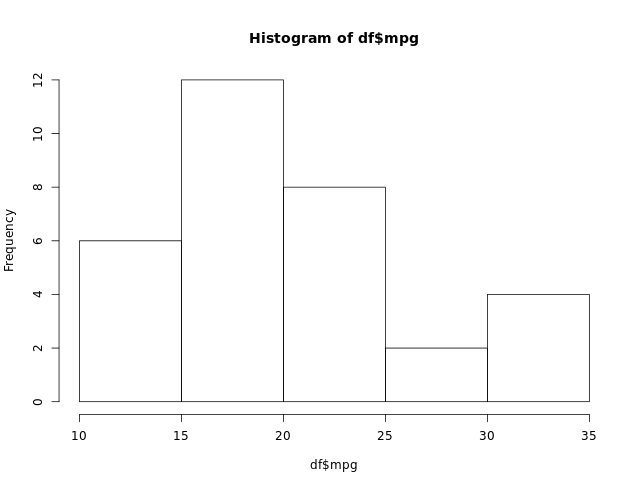hp_plot <- hist(df\$hp)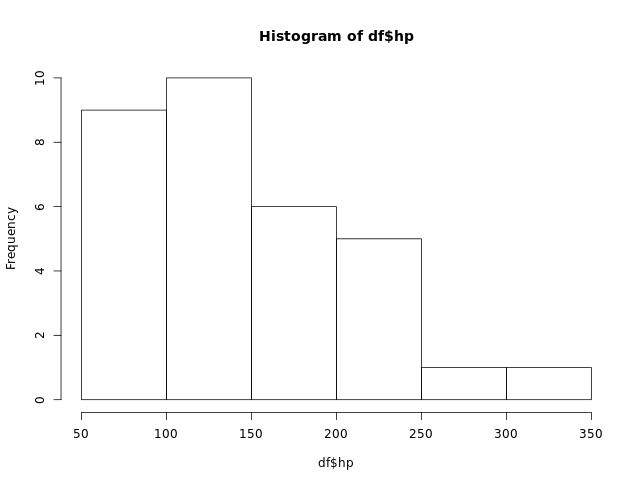drat_plot <- hist(df\$drat)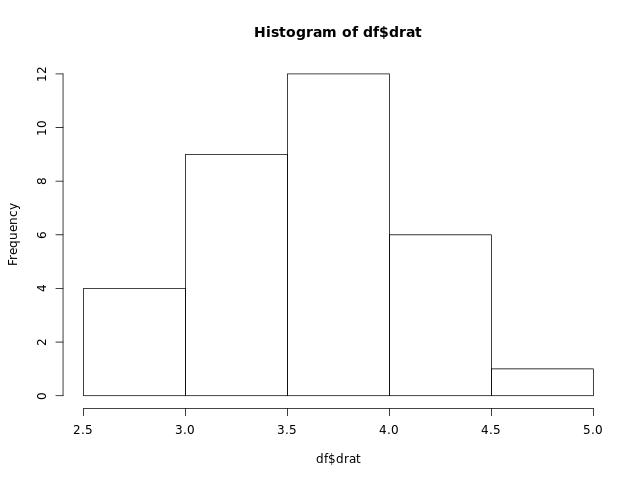```

From above, both mpg and hp look skewed to the right, so we take the log transform of these variables

```mpg_plot <- hist(log(df\$mpg))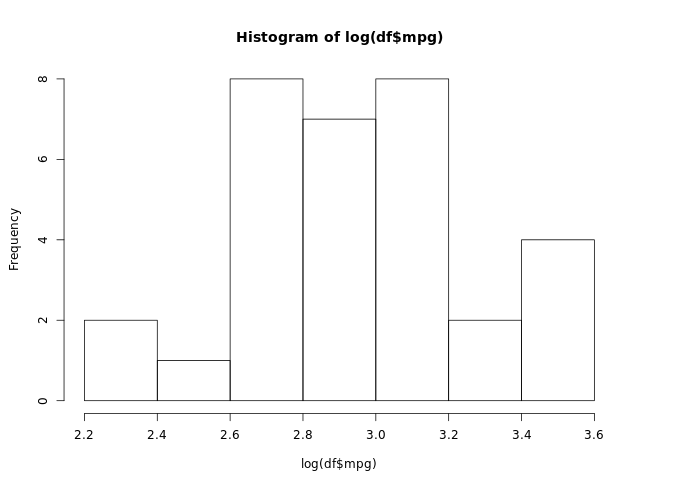hp_plot <- hist(log(df\$hp))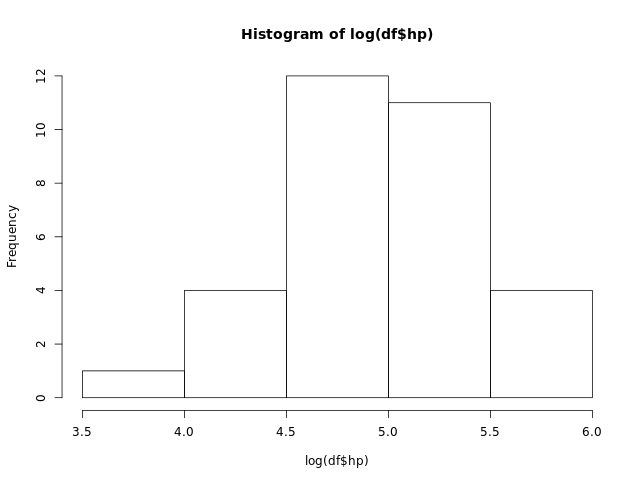```

That looks much better.

Then, split into testing and training data! Note that we always want to do this to ensure we save a subset of our data for additional validation tests on our model.

```df\$test_data_flag <- rbinom(nrow(df), 1, 0.3)
training <- filter(df, test_data_flag == 0)
test <- filter(df, test_data_flag == 1)```

Part 2: Generating the Model

Now we generate the model! This is very similar to generating a bivariate linear regression, only difference is we add a + sign to include more variables in our model.

`model <- lm(log(mpg) ~ log(hp) + drat, data=df)`

To view the slopes we use this command (we avoid using the summary() command for multivariable regressions to ensure our error terms are robust to heteroscedasticity. More on this later). The column under estimate tells us the effect of one variable on the other. Here, a unit change in log(hp) leads to a decrease in 0.43 of log(mpg) (aka, a 43% drop in mpg). The last column Pr(>|t|) lets us know how confident we are in claiming the explanatory variable has any effect on the response variable. Here, we see that the coefficient for log(hp) is significant at the 0.001 level, and drat is not significant at all.

`print(coeftest(model, vcov = vcovHC))`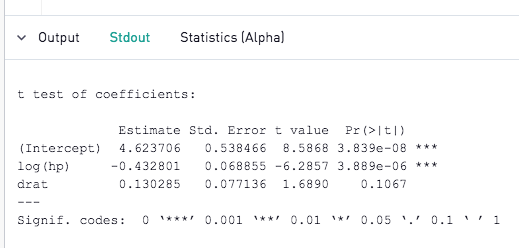To assess goodness of fit of the whole model, we take a look at the adjusted R squared value. Note that we look at the adjusted R squared instead of the regular R squared to account for the fact that we have multiple variables. This is because R squared will always increase as you add more variables, even if they do not contribute to response variable. Adjusted R squared takes this into account and “penalizes” additional variables.

`print(summary(model)\$adj.r.squared)`

This gives us an adjusted r-squared value of about 0.76. Remember, the closer this value is to 1, the better the model is at predicting y.

Part 3: Evaluating the Model

Now to evaluate our model, we check that we have met the following conditions.

Condition 1: Is there linearity? Note that by generating the model itself, we are forcing this condition. Our later conditions will constrain this further to something more tangible!

Condition 2: Is the sample random? This requires background knowledge of your sample. An easy way this gets violated is by clustering. Ex: Rather than randomly sampling 1000 students, I randomly select 100 classes and take 10 students from each. This clustering effect violates the random assumption, so we cannot use the model to make any claims based on inference

Condition 3: Verify there are no cases of perfect multicollinearity between variables. In other words, if I have weight in pounds and weight in kgs as two variables in a model, this would lead to inaccuracies as the two are essentially the same thing. Even if variables aren’t perfectly correlated, it does reduce the precision of our model if they are highly correlated. To test this, run the vif() command on the model as shown below and view the output in the stdout tab. As best practice, values above 4 signify variables that are highly correlated with another variable. To remedy this, consider dropping one of the highly multicollinear variables from your regression model!

`print(vif(model))`

In our example here, vif for both variables is under 2, so we meet this condition.

Condition 4: Ensure there is no trend in the residual plot. Residuals are the difference between the predicted and the actual values. In a bivariate regression, we plot the residuals against the explanatory variable x. For a multivariable regression, like the one here, plot the residuals against the predicted y values (this is more efficient and capture interactions between the explanatory variables better than plotting residuals against every explanatory variable in your model!). We can quickly pull this plot from r from the following command: plot(model, which = 1). In this plot, we want the red line to be as flat as possible

```plot(model, which = 1)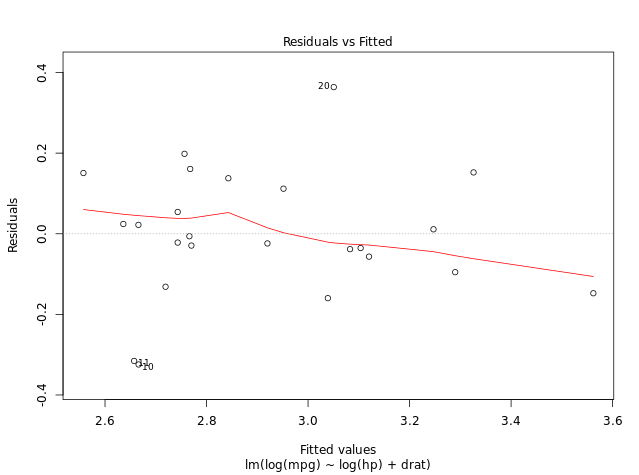```

Given the small sample size, the line is actually pretty rough here. However, you should aim for a flatter line in your models with larger samples!

Condition 5: Verify that the variance in the residuals is consistent. In other words, there should be the same amount of "scatter" moving left to right in the above chart. Another way of assessing this criteria is by checking that the red line in plot(model, which = 3) is as flat as possible. If there is variance in the “thickness” of the band formed by all the residuals, we say the model is heteroscedastic. This doesn’t necessarily mean our model is bad, but it means we need to use a different set of standard errors than those presented in the summary(model) command. This is why we used the coeftest(model, vcov = vcovHC) earlier. Note, unless you are absolutely confident your model has equal variance in the residuals (is homoscedastic), we recommend using the heteroscedastic-robust errors from the coeftest(model, vcov = vcovHC)  command.

```plot(model, which = 3)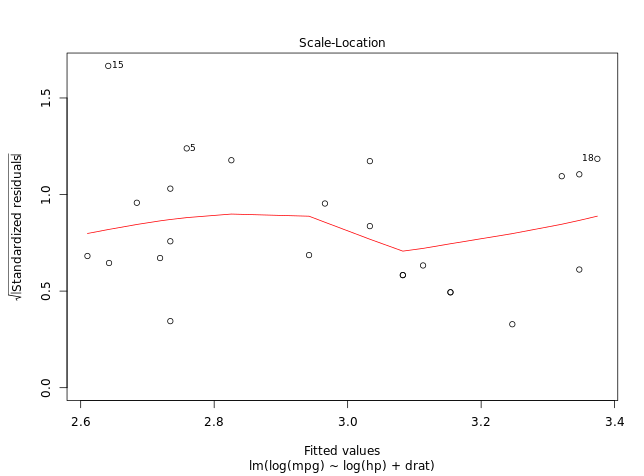```

Condition 6: Normality of residuals - we want to ensure the distribution of error terms is normal. We can do this in 2 ways. (1) run the command plot(model, which = 2) and assess whether the points match the dotted line as close as possible, or 2. Run plot(model.residuals) and assess whether the histogram looks like a bell curve

```plot(model, which = 2)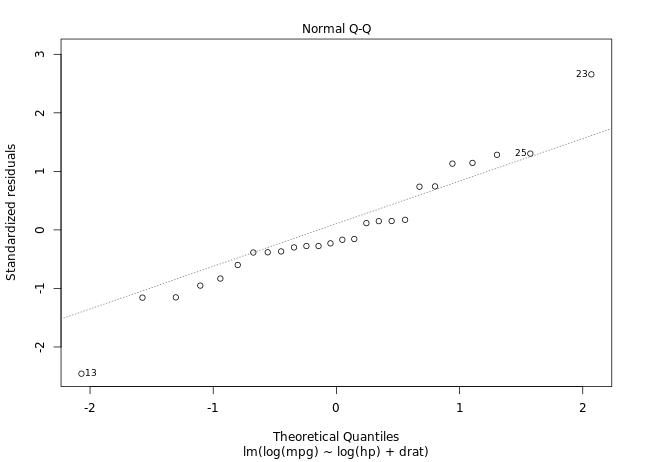hist(model\$residuals)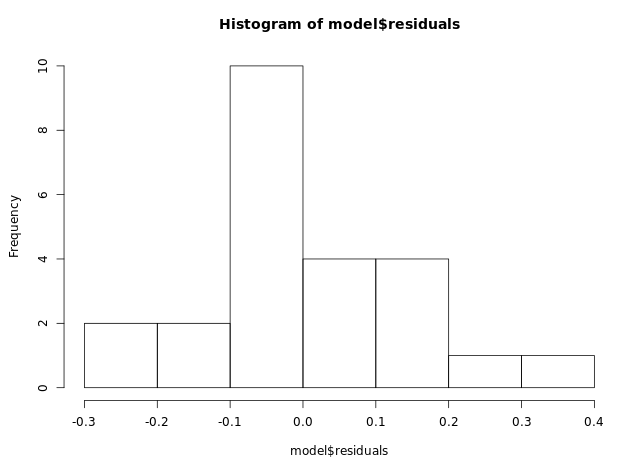```

A note on outliers: Lastly, we want to understand if any outliers are driving the results of the model. We can uncover this using the plot(model, which=5) command. This gives us a residuals vs leverage plot. You can read more details here if you’re interested in learning more, but essentially we are looking for values in the top right and bottom right regions outside of the red boundary. This signifies that the data point is an outlier. Note we don’t necessarily want to remove the outlier unless it’s an unrealistic value. However, knowing they exist is important context in the model generation process

```plot(model, which = 5)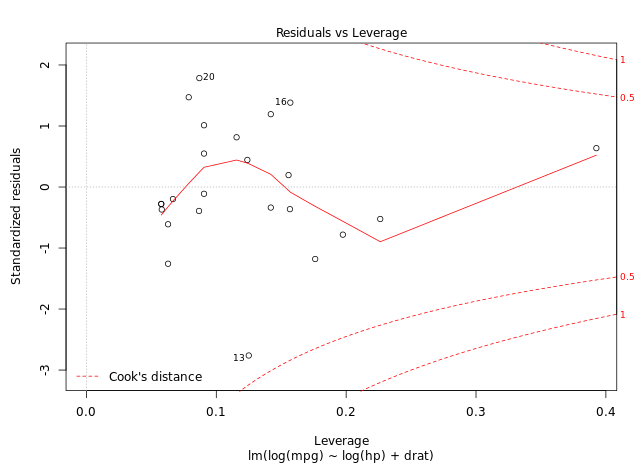```

Part 4: Validating the Model against the Test Data

Note, all these above steps are done on the same dataset used to train the data. However, it is always a good idea to check how these conditions hold against the testing dataset as well. We show how to do this below.

Assuming you have randomly assigned values into your testing and training dataset, we can assume that Conditions 1,2,3 on the testing dataset are in line with the training dataset. Therefore, let's jump to Conditions 4 and 5. We run the following command to get a column of predicted y values using our model on the test dataset.

`test\$predicted.mpg <- predict(model, test)`

Pass this table into periscope.table as shown below

`periscope.table(test)`

Plotting the predicted values against the residuals as a scatter plot using the Periscope UI and adding a trendline gives the following residual plot: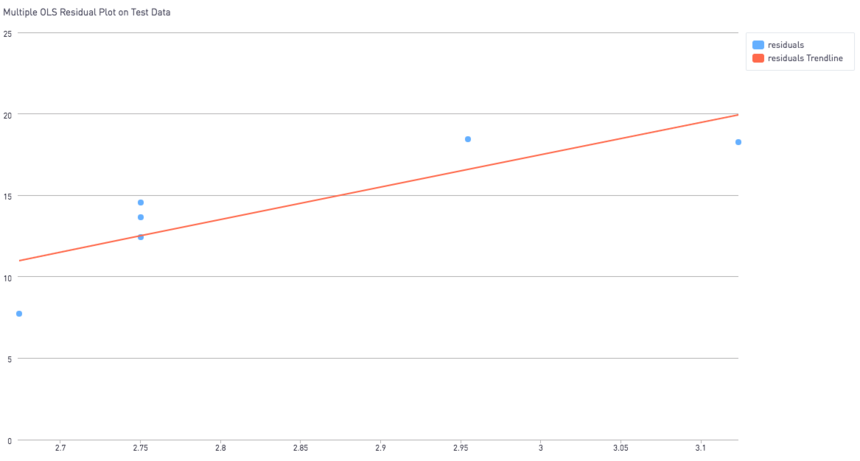Since this data frame is pretty small, we don’t have very many values in the testing dataset, so this particular example is admittedly not that insightful here.

For Condition 6, the distribution of residuals, we comment out the periscope.table(test) line and run the following command

```residual_distribution <- hist(test\$predicted.mpg)
periscope.image(residual_distribution)```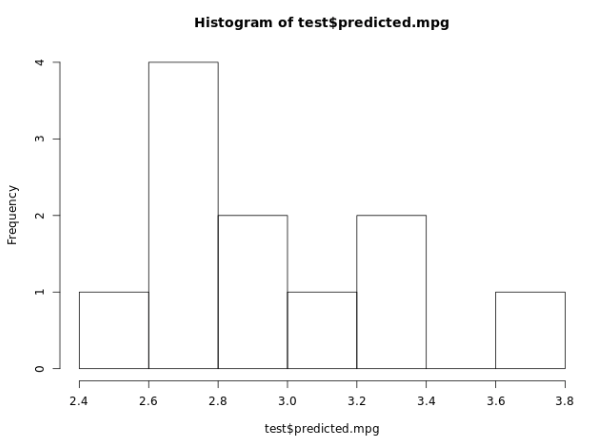Remember, we want this distribution to be as bell-curved as possible, but given the small sample size, any such pattern is hard to see in this particular example

If you made it this far and all of your tests have checked out, congratulations! You have a robust regression model that can be used for inference, with the statistics to back it :)

Want something above elaborated further? Post in the comments below, and I may write a follow up post addressing FAQs ;)